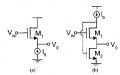# cascode non dominant pol

#### mirror_pole

Joined Nov 14, 2020
8
Hey guys
I came across the FVF circuit (circuit b) and there is one thing i dont get. If i analyse the open loop configuration by cutting the feedback, setting Vin=0 and applying a V,test at the gate of M2 i get a regular cascode configuration with a current source load Ib. Concidering the small signal model and assuming that my V,test is an ideal small signal voltage source (therefore Rs=0) i can say that my dominant pol is assosiated with the output node while the non dominant pol is at the source of M1 (approximation!).

Reading the following paper https://ieeexplore.ieee.org/document/1487657 the autors say that one can determine pnd by the product of the resistance seen at the source node of M1 and the sum of the caps.
Resistance is pretty straight forward: R,source,M1=r02//((rb+r01)/(gm1ro1+1)) where rb is the res of the current source.

And this is the part that i dont get. If i approach the pol assosiated with that node my cap at the output shunts the current source Ib at higher frequencies simply because of the dominant pol and therefore M1 acts as a diode connected device independant of cascode load!
Therefore the non dominant pol of the cascode is the dominant pol of the common source stage M2 with diode connected load M1 and i simply dont get why they consider the current source load for determination of the non dominant pol.

I also did an exact calculation with Rosenstark method, calculating the return ratio whichs actually the same as cutting the feedback loop and applying a V,test. Using some approximations like large self gain, magnitude of the caps and assuming pd>>pnd i can associate the dominant pol exactly with the output node but as for pnd approximatly gm1/Cx where Cx is the sum of the caps at the source of M1 and which actually fits the argumentation above (in this case R,source,M1=ro2//ro1//1/gm1 approximatly 1/gm1 assuming gm,i*ro,j>>1)
So yeah i would really like to know why they consider the full resistance to calculate pnd.

Thanks guys